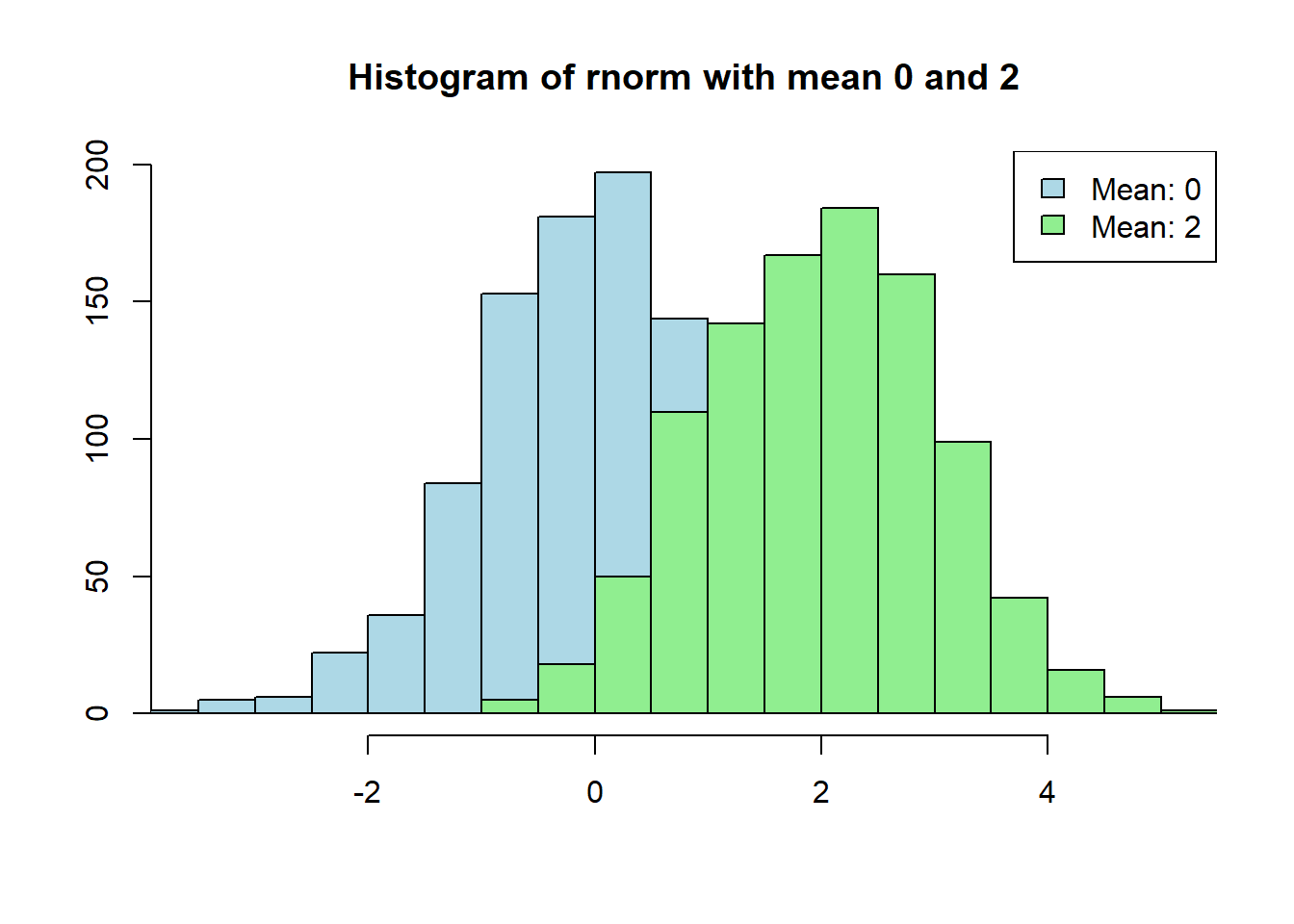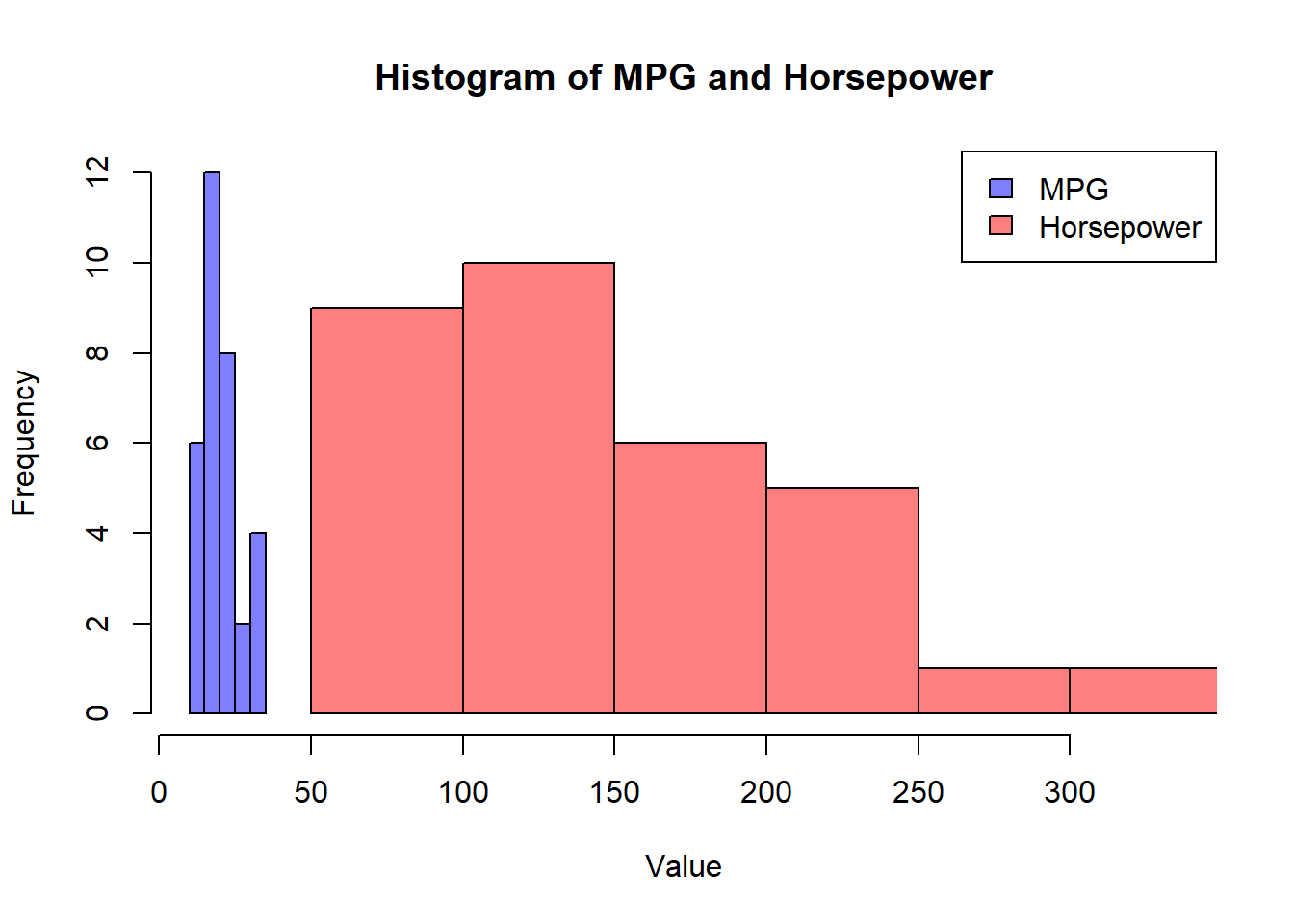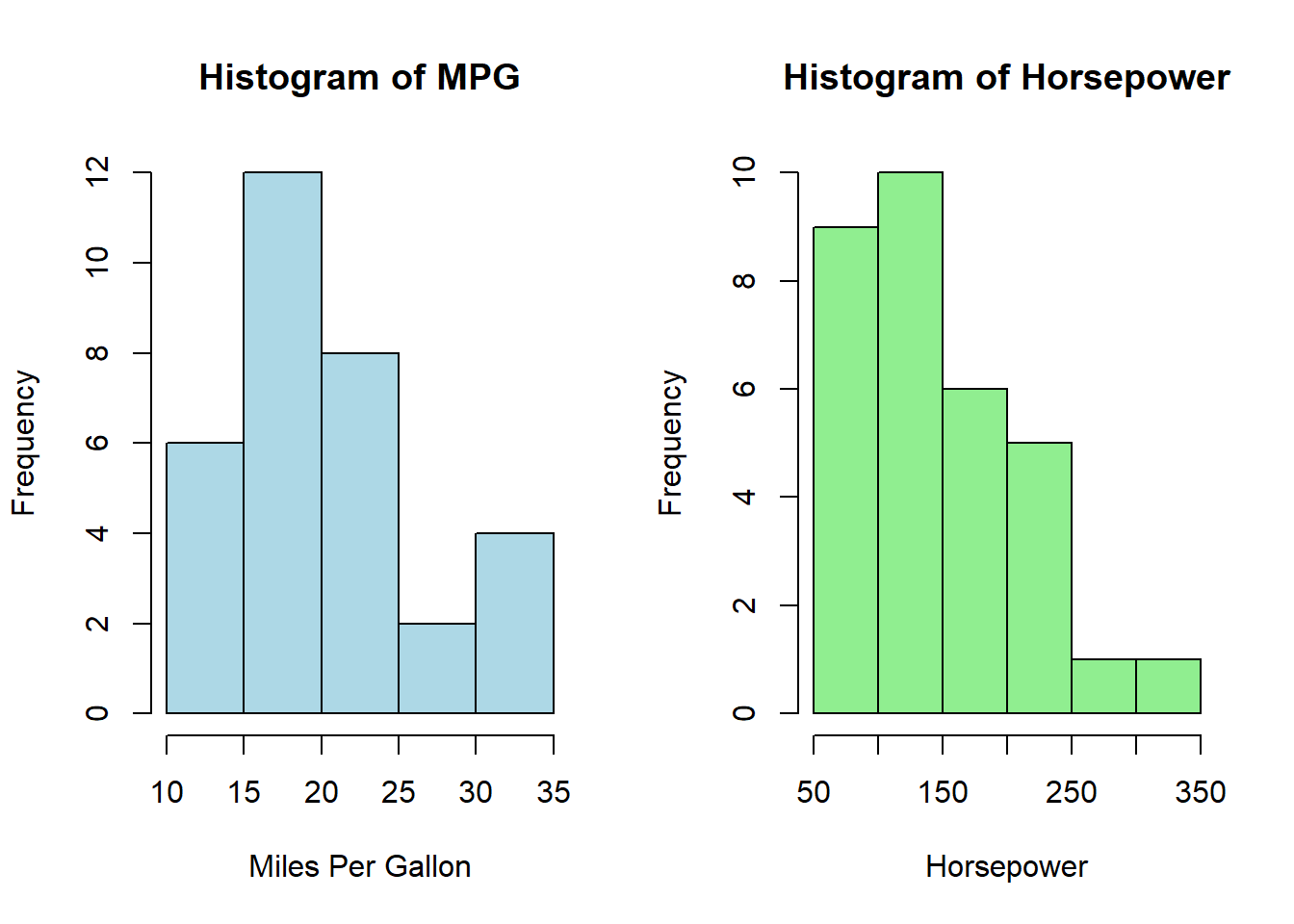# Histograms with Two or More Variables in R

rtip
viz
Author

Steven P. Sanderson II, MPH

Published

September 15, 2023

# Introduction

Histograms are powerful tools for visualizing the distribution of a single variable, but what if you want to compare the distributions of two variables side by side? In this blog post, we’ll explore how to create a histogram of two variables in R, a popular programming language for data analysis and visualization.

We’ll cover various scenarios, from basic histograms to more advanced techniques, and explain the code step by step in simple terms. So, grab your favorite dataset or generate some random data, and let’s dive into the world of dual-variable histograms!

# Prerequisites

Before we start, ensure you have R installed on your computer. You can download it from R’s official website. Additionally, you might find it helpful to have RStudio, an integrated development environment for R.

# Examples

## Basic Dual-Variable Histogram

Let’s begin with the most straightforward scenario: creating a histogram of two variables using the `hist()` function. We’ll use the built-in `mtcars` dataset, which contains information about various car models.

``````x1 <- rnorm(1000)
x2 <- rnorm(1000, mean = 2)
minx <- min(x1, x2)
maxx <- max(x1, x2)

# Create a basic dual-variable histogram
hist(x1, main="Histogram of rnorm with mean 0 and 2", xlab="",
ylab="", col="lightblue", xlim = c(minx, maxx))
hist(x2, xlab="",
legend("topright", legend=c("Mean: 0", "Mean: 2"), fill=c("lightblue", "lightgreen"))``````The given R code generates a dual-variable histogram in R using the `hist()` function. The first two lines of code generate two vectors `x1` and `x2` of 1000 random normal numbers each, with `x1` having a mean of 0 and `x2` having a mean of 2. The `min()` and `max()` functions are then used to find the minimum and maximum values between `x1` and `x2`. These values are used to set the limits of the x-axis of the histogram.

The `hist()` function is then called twice to create two histograms, one for `x1` and one for `x2`. The `col` argument is used to set the color of each histogram. The `add` argument is set to `TRUE` for the second histogram so that it is overlaid on top of the first histogram. Finally, the `legend()` function is used to add a legend to the plot indicating which histogram corresponds to which variable.

In summary, the code generates a dual-variable histogram of two vectors of random normal numbers with different means. The histogram shows the distribution of values for each variable and allows for easy comparison between the two variables.

## Dual-Variable Histogram with Transparency

Adding transparency to the histograms can make the visualization more informative when the bars overlap. We can achieve this by setting the `alpha` parameter in the `col` argument. Let’s use the same dataset and create a dual-variable histogram with transparency:

``````# Create a dual-variable histogram with transparency
minx <- min(mtcars\$mpg, mtcars\$hp)
maxx <- max(mtcars\$mpg, mtcars\$hp)
hist(
mtcars\$mpg,
main="Histogram of MPG and Horsepower",
xlab="Value",
ylab="Frequency",
col=rgb(0, 0, 1, alpha=0.5),
xlim=c(minx, maxx))
hist(
mtcars\$hp,
col=rgb(1, 0, 0, alpha=0.5),
)
legend("topright", legend=c("MPG", "Horsepower"), fill=c(rgb(0, 0, 1, alpha=0.5), rgb(1, 0, 0, alpha=0.5)))``````Here, we use the `rgb()` function to set the color with transparency. The `alpha` parameter controls the transparency level, with values between 0 (completely transparent) and 1 (completely opaque).

## Side-by-Side Histograms

If you prefer to display the histograms side by side, you can use the `par()` function to adjust the layout. Here’s an example:

``````# Set up a side-by-side layout
par(mfrow=c(1, 2))

# Create side-by-side histograms
hist(mtcars\$mpg, main="Histogram of MPG", xlab="Miles Per Gallon",
ylab="Frequency", col="lightblue", xlim=c(10, 35))
hist(mtcars\$hp, main="Histogram of Horsepower", xlab="Horsepower",
ylab="Frequency", col="lightgreen")````````par(mfrow=c(1,1))``

In this code, we use `par(mfrow=c(1, 2))` to set up a 1x2 layout, which means two plots will appear side by side.

## Customizing Dual-Variable Histograms

You can customize your dual-variable histograms further by adjusting various parameters, such as bin width, titles, labels, and colors. Experiment with different settings to create visualizations that best convey your data’s story.

Remember, the key to effective data visualization is experimentation and exploration. Try different datasets, play with colors and styles, and find the representation that best suits your needs.

## Conclusion

In this blog post, we’ve explored several ways to create histograms of two variables in R. Whether you’re comparing distributions or just visualizing your data, histograms are a valuable tool in your data analysis toolkit. Experiment with the provided examples and take your data visualization skills to the next level!

So, fire up your R environment, load your data, and start creating dual-variable histograms today. Happy coding!